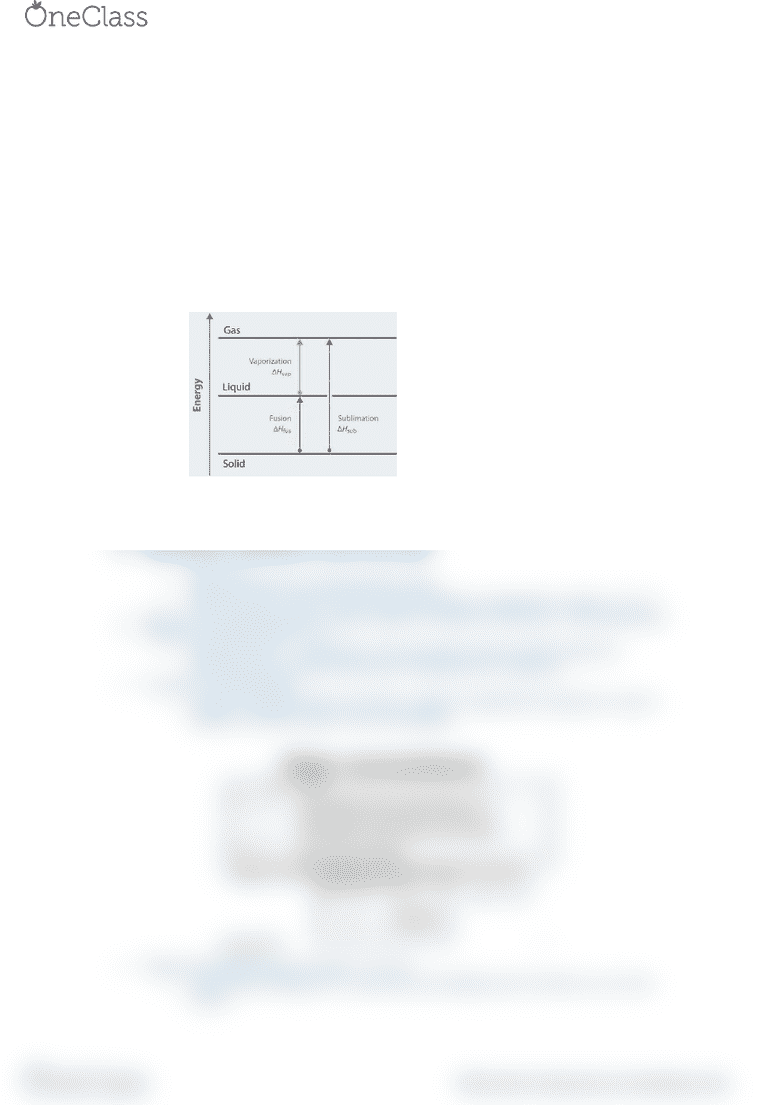Textbook Notes (280,000)
US (110,000)
CHM (9)
Chapter 6

# CHM 111 Chapter Notes - Chapter 6: Letter Case, Ionic Compound, Heat Capacity

This preview shows half of the first page. to view the full 2 pages of the document.CHAPTER 6
Energy is the capacity to do work
o Work is directed energy change resulting from a process
o Thermal Energy: random motion of atoms and molecules
o Chemical Energy: stored within structural units of chemical substances
o Potential Energy: energy available by virtue of object’s position
Law of Conservation of Energy: total quantity of energy in the universe is assumed constant
Heat: transfer of thermal energy b/w 2 bodies that are at different temperatures
Thermochemistry: study of heat change in chemical reactions
o Open system: can exchange mass and energy (usually in form of heat w/
surroundings)
o Closed system: transfer of heat (energy) but not mass
o Isolated system: no mass or energy transfer
Exothermic process: process that gives off heat to surroundings
Endothermic process: process where surroundings supply heat to the system
Thermodynamics: study of interconversion of heat
State functions: determined by state of the system
o E, P, V, T
o Magnitude based on initial and final values
o State of system: values of macroscopic properties (composition, energy, T, P, V)
First Law of Thermodynamics: energy cannot be created nor destroyed - only transformed
Change in internal energy: U=Uf-Ui
o Internal energy - 2 components: KE (molecular motion) and PE (attractive
interactions b/w electrons and nuclei, interactions b/w molecules
Usystem= -Usurroundings
o Change in internal energy of system is equal in magnitude but opposite in sign to
change in internal energy of the surroundings
Change in internal energy of system = U=q+w
o Sum of heat exchange b/w system and surroundings and work done on or by the
system
find more resources at oneclass.com
find more resources at oneclass.com